# MCQ in AC Circuits Part 7 | REE Board Exam

(Last Updated On: November 13, 2020)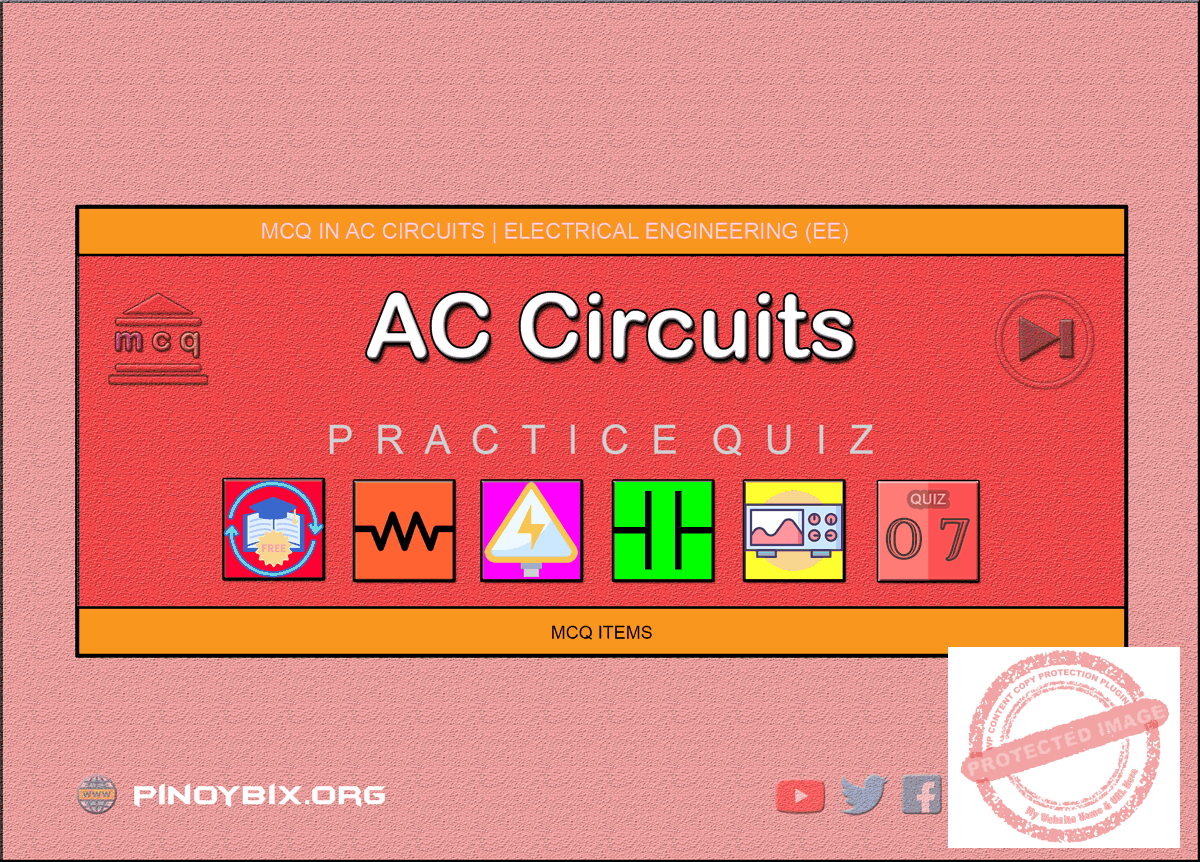This is the Multiple Choice Questions Part 7 of the Series in AC Circuits as one of the Electrical Engineering topic. In Preparation for the REE Board Exam make sure to expose yourself and familiarize in each and every questions compiled here taken from various sources including but not limited to past Board Exam Questions in Electrical Engineering field, Electrical Engineering Books, Journals and other Electrical Engineering References.

#### Continue Practice Exam Test Questions Part 7 of the Series

SERIES CIRCUITS

Choose the letter of the best answer in each questions.

301.  A circuit component that opposes the change in circuit voltage is

A.  resistance

B.  capacitance

C.  inductance

D.  all of these

Solution:

302.  Power loss in an electrical circuit can take place in

A.  inductance only

B.  capacitance only

C.  inductance and resistance

D.  resistance only

Solution:

303.  A circuit of zero lagging power factor behaves as

A.  an inductive circuit

B.  a capacitive circuit

C.  R-L circuit

D.  R-C circuit

Solution:

304.  In an R-L series circuit the power factor is

B.  lagging

C.  zero

D.  unity

Solution:

305.  When a sinusoidal voltage is applied across an R-L series circuit having R = XL, the phase angle will be

A.  90°

B.  45° lagging

Solution:

306.  An  ac  source  having  voltage  e  =  110  sin  (ωt  +  π/3)  is  connected  in  an  ac circuit. If the current drawn from the circuit varies as i = 5 sin (ωt  –  π/3) the impedance of the circuit will be

A.  22 Ω

B.  16 Ω

C.  30.8   Ω

D.  none of these

Solution:

307.  Which are of the following true of the circuit shown in the given figure?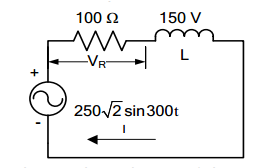1.  VR =  100√2  V        2.  I = 2 A      3.  L = 0.25 H

Select the correct answer using the codes given below:

Codes:

A.  2 and 3

B.  1 and 2

C.  1 and 3

D.  1, 2 and 3

Solution:

308.  The  R-L  circuit  of  the  figure  is  fed  from  a  constant  magnitude  variable frequency  sinusoidal  voltage  source  vin.  At  100  Hz,  the  R  and  L  element each has a voltage drop Vrms. If the  frequency of the source is changes to 50 Hz, then new voltage drop across R is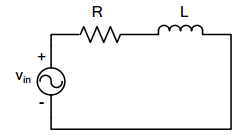A.  √(5/8) Vrms

B.  √(2/3) Vrms

C.  √(8/5) Vrms

D.  √(3/2) Vrms

Solution:

309.  An  ac  source  of  200  Vrms  supplies  active  power  of  600  W  and  reactive power of 800 VAR. The rms current drawn from the source is

A.  10 A

B.  5 A

C.  3.75 A

D.  2.5 A

Solution:

310.  A square wave is fed to an R-C circuit. Then

A.  voltage across R is square and across C is not square

B.  voltage across C is square and across R is not square

C.  voltage across both R and C is square

D.  voltage across both R and C is not square

Solution:

311.  The  voltage  phasor  of  a  circuit  is 10∠15° V  and  the  current  phasor  is 2∠-45° A. The active and reactive powers in the circuit are

A.  10 W and 17.32 VAR

B.  5 W and 8.66 VAR

C.  20 W and 60 VAR

D.  20√2 W and  10√2 VAR

Solution:

312.  In a two-element series circuit, the applied voltage and resultant current are respectively, v(t) = 50 + 50 sin (5 x 103 t) and i(t) = 11.2 sin (5 x 103 t + 63.4°). The nature of the elements would be

A.  R-L

B.  R-C

C.  L-C

D.  neither R, nor L, nor C

Solution:

313.  A  series  circuit  passive  elements  has  the  following  current  and  applied voltage: v = 200 sin (2,000t + 50°), i = 4 cos (2,000t + 13.2°). The circuit elements

A.  must be resistance and capacitance

B.  must be resistance and inductance

C.  must be inductance, capacitance and resistance

D.  could  be  either  resistance  and  capacitance  or  resistance,  inductance and capacitance

Solution:

314.  A two terminal black box contains one of the R-L-C elements. The black box is connected to a 220 V ac supply. The current through the source is I. When a capacitance of 0.1 F is inserted in series between the source and the box, the current through the source is 2I. The element is

A.  a resistance

B.  an inductance

C.  a capacitance

D.  it is not possible to determine the element

Solution:

315.  In the following circuit, i(t) under steady state is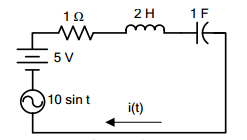A.  zero

B.  5

C.  7.07 sin t

D.  7.07 sin (t – 45°)

Solution:

316.  The source in the circuit is a sinusoidal source. The supply voltages across various elements are marked in the figure. The input voltage is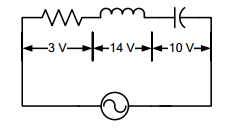A.  10 V

B.  5 V

C.  27 V

D.  24 V

Solution:

317.  In the circuit shown in the given figure, if the power consumed by the 5 Ω resistor is 10 W, then the pf of the circuit is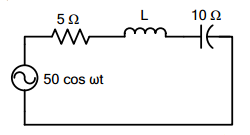A.  0.8

B.  0.6

C.  0.5

D.  zero

Solution:

318.  In  an  RL  circuit,  supplied  from  an  ac  source,  the  reactive  power  is proportional to the

A.  the average energy stored in the electric field

B.  the average energy stored in the magnetic field

C.  sum of the average energy stored in the electric field and that stored in the magnetic field

D.  difference  of  the  average  energy  stored  in  the  electric  field  and  that stored in the magnetic field

Solution:

319.  If a series RLC circuit excited by a voltage e = E sin ωt when LC < 1/ω2

A.  current lags behind the applied voltage

B.  current leads the applied voltage

C.  current is in phase with the applied voltage

D.  voltage across L and C are equal

Solution:

320.  The current in the circuit shown is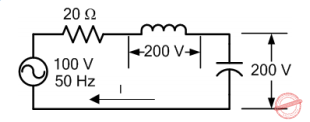A.  5 A

B.  10 A

C.  15 A

D.  25 A

Solution:

321.  In the case of the R-L-C circuit shown in the given figure, the voltage across the R, L and C would be respectively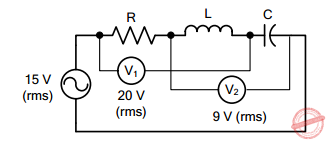A.  12 V, 16 V and 7 V or 25 V

B.  16 V, 12 V and 7 V or 25 V

C.  7 V, 16 V and 12 V

D.  16 V, 12 V and 25 V

Solution:

322.  Consider the following statements regarding the circuit shown in the figure.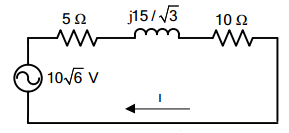If the power consumed by 5 Ω resistor is 10 W then

1.  |I| = √2  A

2.  the total impedance of the circuit is 5 Ω

3.  cos θ = 0.866

Which of these statements is correct?

A.  1 and 3

B.  2 and 3

C.  1 and 2

D.  1, 2 and 3

Solution:

323.  In an ac circuit if voltage V = (a + jb) and current I = (c + jd), then the power is given by

B.  ac + bd

Solution:

324.  The reactive power drawn from the source in the network in the given figure is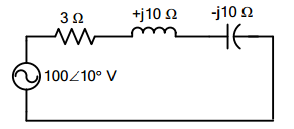A.  300 VAR

B.  200 VAR

C.  100 VAR

D.  zero

Solution:

325.  A  series  R-L-C  circuit,  consisting  of  R  =  10  Ω,  XL  =  20  Ω,  XC  =  20  Ω  is connected  across  an ac supply  of  100  V  (rms).  The  magnitude  and  phase angle (with reference to supply voltage) of the voltage across the inductive coil are respectively

A.  100 V, 90°

B.  100 V, -90°

C.  200 V, -90°

D.  200 V, 90°

Solution:

326.  For a capacitor in a sine wave ac circuit

A.  vC lags iC by 90°

B.  iC leads vC by 90°

C.  iC and vC have the same frequency

D.  all of these

Solution:

327.  In a series RC circuit,

A.  VC leads VR by 90°

B.  VC and I are in phase

C.  VC lags VR by 90°

D.  both B and C

Solution:

328.  In a series RC circuit,

A.  VC and VR are in phase

B.  VT and I are always in phase

C.  VR and I are in phase

D.  VR leads I by 90°

Solution:

329.  When the frequency of the applied voltage increases in a series RC circuit

A.  the phase angle, θT, becomes more negative

B.  ZT increases

C.  ZT decreases

D.  both A and B

Solution:

330.  Inductive reactance, XL

A.  applies only to non-sinusoidal waveforms or dc

B.  applies only to sine waves

C.  applies to either sinusoidal or non-sinusoidal waveforms

D.  is inversely proportional to frequency

Solution:

331.  For an inductor in a sine wave ac circuit

A.  VT leads iL by 90°

B.  VT lags iL by 90°

C.  VT and iL are in phase

D.  none of these

Solution:

332.  In a series RL circuit,

A.  VT lags VR by 90°

B.  VT leads VR by 90°

C.  VR and I are in phase

D.  both B and C

Solution:

333.  In a series RL circuit where XL = R, the phase angle, θZ, is

A.  -45°

B.  0°

C.  90°

D.  45°

Solution:

334.  In an ac circuit with only series resistances

A.  VT and I are in phase

B.  RT =R1 + R2 + R3 + … + etc.

C.  each voltage drop is in phase with the series current

D.  all of these

Solution:

335.  The unit of apparent power is the

A.  volt-ampere (VA)

B.  watt (W)

C.  volt-ampere-reactive (VAR)

D.  joule (J)

Solution:

336.  In an ac circuit with only series capacitors

A.  VT leads I by 90°

B.  VT lags I by 90°

C.  each capacitor voltage drop leads I by 90°

D.  both A and C

Solution:

337.  The unit of real power is the

A.  watt (W)

B.  volt-ampere (VA)

C.  joule (J)

D.  volt-ampere-reactive (VAR)

Solution:

338.  In a series RLC circuit

A.  XL and XC are 180° out of phase

B.  IL and IC are 180° out of phase

C.  XL and XC are 90° out of phase

D.  XL and XC are in phase

Solution:

339.  The power factor of an ordinary electric bulb is

A.  zero

B.  unity

C.  slightly more than unity

D.  slightly less than unity

Solution:

340.  The power factor of an ac circuit is equal to

A.  cosine of the phase angle

B.  sine of the phase angle

C.  unity for a resistive circuit

D.  unity for a reactive circuit

Solution:

341.  If  f(t)  =  sin  t  +  sin  √2  t  is  passing  through  R  =  1  ohm,  what  is  the  power dissipated in 1 ohm resistor?

A.  1 W

B.  2 W

C.  since f(t) in non-periodic, not possible to find power

D.  none of these

Solution:

C.  PARALLEL CIRCUITS

342.  EE Board Exam October 1981

A  circuit  consists  of  XL  =  j5  ohms,  XC  =  -j5  ohms  and  R  =  5  ohms  all  are connected in parallel. Find the equivalent impedance.

A.  5.5 Ω

B.  5.0 Ω

C.  4.8 Ω

D.  5.2 Ω

Solution:

343.  EE Board Exam October 1985

Given: Z1  =  -j2.5 ohms; Z2  = j4 ohms; Z3  = 5 ohms; Z = 1 + j5 ohms. If the four impedances are connected in parallel, find the equivalent impedance in ohms.

A.  4.1 + j0.72

B.  4.3 + j0.45

C.  4.2 + j0.35

D.  4.0 + j0.97

Solution:

344.  EE Board Exam April 1984, April 1987

Three impedances Za = 3 + j4 ohms, Zb = 4 –  j4 ohms  and Zc = j3 ohms are connected in parallel. Solve for the pf of the combination.

B.  0.554 lagging

D.  0.620 lagging

Solution:

345.  EE Board Exam October 1993

A pure capacitance of 530.515 x 10-6 farad and an inductance of 530.515 x 10-4 Henry  are  connected  in parallel  across  an ac  power  source.  Solve  for the resultant impedance assuming that the frequency is 30 Hz.

A.  10 Ω

B.  infinite

C.  zero

D.  undefined

Solution:

346.  REE Board Exam March 1998

A  coil  of  a  50-ohm  resistance  and  of  150  mH  inductance  is  connected  in parallel with a 50 μF capacitor. What is the power factor of the circuit?

A.  80%

B.  50%

C.  70%

D.  60%

Solution:

347.  EE Board Exam April 1982

Three impedances Za, Zb and Zc are connected in parallel. If at 60 Hz, Za = j8, Zb = -j2 and Zc = 5 ohms. Solve for the resultant power factor.

A.  0.471 lagging

C.  0.573 lagging

Solution:

348.  REE Board Exam October 1997

A resistor of 50 ohms and an impedance of 100 + j50 ohms are connected in parallel across a 220 volts supply. What is the power factor of the load?

A.  96%

B.  99%

C.  98%

D.  95%

Solution:

349.  EE Board Exam October 1992

A capacitor of 3.18 microfarads is connected in parallel with a resistance of 2,000  ohms.  The  combination  is  further  connected  in  series  with  an inductance of 795 mH and  resistance of 100 ohms across a supply given by e = 400 sin wt + 80 sin (3wt + 60°). Assume w = 314 radians/sec. Determine the power dissipated.

A.  74.66 W

B.  78.05 W

C.  80.28 W

D.  75.66 W

Solution:

350.  EE Board Exam October 1992

A capacitor of 3.18 microfarads is  connected in parallel with a resistance of 2,000  ohms.  The  combination  is  further  connected  in  series  with  an inductance of 795 mH and resistance of 100 ohms across a supply given by e = 400 sin wt + 80 sin (3wt + 60°). Assume w = 314 radians/sec. Determine the circuit power factor.

A.  0.702

B.  0.650

C.  0.633

D.  0.612

Solution:

#### Questions and Answers in AC Circuits

Following is the list of practice exam test questions in this brand new series:

MCQ in AC Circuits
PART 1: MCQ from Number 1 – 50                  Answer key: included
PART 2: MCQ from Number 51 – 100              Answer key: included
PART 3: MCQ from Number 101 – 150            Answer key: included
PART 4: MCQ from Number 151 – 200            Answer key: included
PART 5: MCQ from Number 201 – 250             Answer key: included
PART 6: MCQ from Number 251 – 300             Answer key: included
PART 7: MCQ from Number 301 – 350             Answer key: included
PART 8: MCQ from Number 351 – 400             Answer key: included
PART 9: MCQ from Number 401 – 450             Answer key: included
PART 10: MCQ from Number 451 – 500           Answer key: included

P inoyBIX educates thousands of reviewers and students a day in preparation for their board examinations. Also provides professionals with materials for their lectures and practice exams. Help me go forward with the same spirit.

“Will you subscribe today via YOUTUBE?”

Subscribe

PinoyBIX Engineering. © 2014-2020 All Rights Reserved | How to Donate? |